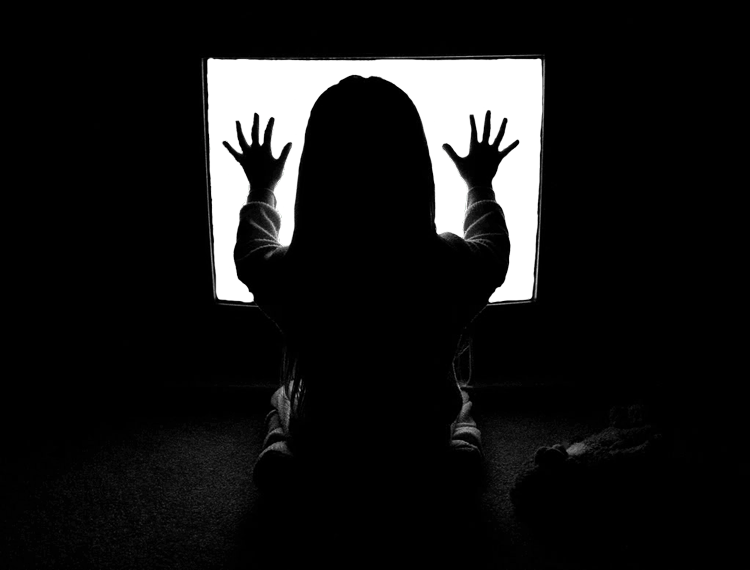# They’re Heeereee: Animating Static in HTML5 Canvas

In last week’s article I talked about making static static in : a single pass of random greyscale blocks in a rectangle. In this piece I will animate that effect with a purpose: to fill in the area of a TV screen in the famous still from Tobe Hooper’s Poltergeist, making an HTML5 “cinemagraph”.

## Setting The Stage

The way in which the static is drawn on the page is slightly odd: instead of occupying the entire area of the `<canvas>` element, the static is drawn in just a portion of that space. The reason will be clear in a moment; first, the HTML markup:

``````<div id="poltergeist">
<canvas id="static" width="750" height="580"></canvas>
</div>``````

And the initial CSS:

``````#poltergeist {
position: relative;
}
#poltergeist canvas, #poltergeist img {
width: 100%;
position: absolute;
top: 0; left: 0;
}``````

The container element uses the standard absolute-inside-relative trick with padding to place the elements on top of each other without displacing other content.

## Getting Static

The static is drawn using a very similar technique to the last article, just in a smaller area:

``````var static = document.getElementById("static"),
context = static.getContext("2d"),
tvHeight = 330,
tvWidth = 550,
pixelWidth = 4,
pixelHeight = 3;

function drawStatic() {
for (var v=55; v < tvHeight; v += pixelHeight){
for (var h=200; h < tvWidth; h += pixelWidth){
lum = Math.floor( Math.random() * 40 );
context.fillStyle = "hsl(0, 0%," + lum + "%)";
context.fillRect(h,v,pixelWidth,pixelHeight);
}
}
requestAnimationFrame(drawStatic);
}
drawStatic();``````

This fills the area where the TV screen will be, measured from the original image in PhotoShop:

Restricting the area of static makes the effect much more efficient.Area of static in canvas, before the PNG is applied on top

Oddly, attempting to paint the PNG file inside the script didn’t work: `<canvas>` would default to using simple transparency for the image, rather than using the full 32-bit range. It was easier to simply place the image on top, adding to the markup:

``````<div id="poltergeist">
<canvas id="static" width="750" height="580"></canvas>
<img src="poltergeist-24.png">
</div>``````

## The Sounds of Static

While not included here, the associated CodePen demo for this article features a “pink noise” sound generated by the Web Audio API to complete the effect:

``````var audioContext = new(AudioContext ||webkitAudioContext),
gainNode = audioContext.createGain(),
bufferSize = 4096,
pinkNoise = (function() {
var b0, b1, b2, b3, b4, b5, b6;
b0 = b1 = b2 = b3 = b4 = b5 = b6 = 0.0;
var node = audioContext.createScriptProcessor(bufferSize, 1, 1);
node.onaudioprocess = function(e) {
var output = e.outputBuffer.getChannelData(0);
for (var i = 0; i < bufferSize; i++) {
var white = Math.random() * 2 - 1;
b0 = 0.99886 * b0 + white * 0.0555179;
b1 = 0.99332 * b1 + white * 0.0750759;
b2 = 0.96900 * b2 + white * 0.1538520;
b3 = 0.86650 * b3 + white * 0.3104856;
b4 = 0.55000 * b4 + white * 0.5329522;
b5 = -0.7616 * b5 - white * 0.0168980;
output[i] = b0 + b1 + b2 + b3 + b4 + b5 + b6 + white * 0.5362;
output[i] *= 0.11;
b6 = white * 0.115926;
}
}
return node;
})();

pinkNoise.connect(audioContext.destination);``````